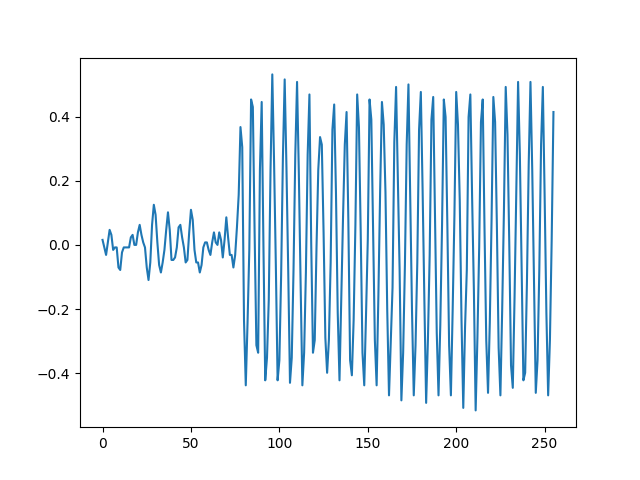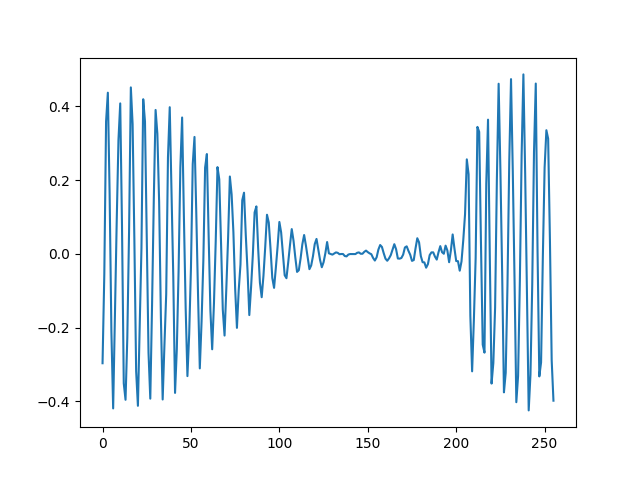# Getting a weired behavior when windowing an audio signal

I am processing audio signals using Essentia, an open-source library and tools for audio and music analysis, description and synthesis. Here is a waveform corresponding to a frame extracted from an audio signal. The plot is obtained with the following instruction:

plt.plot(essentia.array(audio_pool['frames.actual-frames']))... and here is the previous waveform, windowed. The plot is obtained with the conjunction of these two instructions:

w = std.Windowing(type='hamming',
size=len(audio_pool['frames.actual-frames']),
normalized=False)
plt.plot(w(essentia.array(audio_pool['frames.actual-frames'])))We can see that the resulting waveform is somehow inverted, i.e. the beginning of the original signal occurring in the middle.

I tried other window types (rect, hann, etc.) as well as w/ and w/o zero-padding the original signal, I get the same behavior.

It turns out that the windowing function that is used performs by default "Zero-Phase Zero-Padding" on the input signal. You can disable this feature by setting the zeroPhase parameter to False as follows:
w = std.windowing(type='hamming',